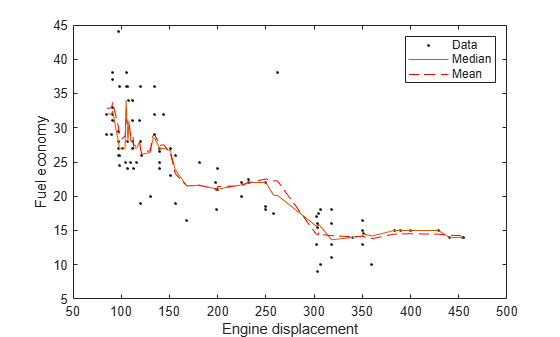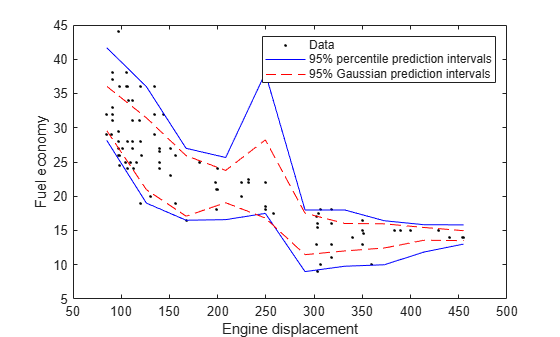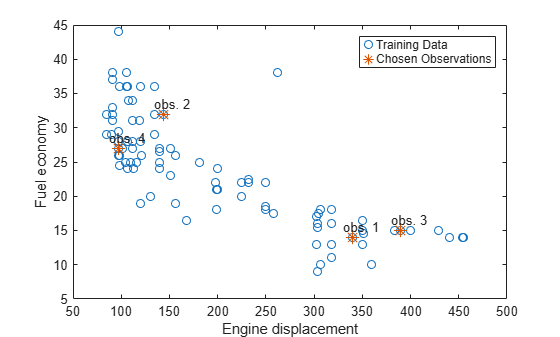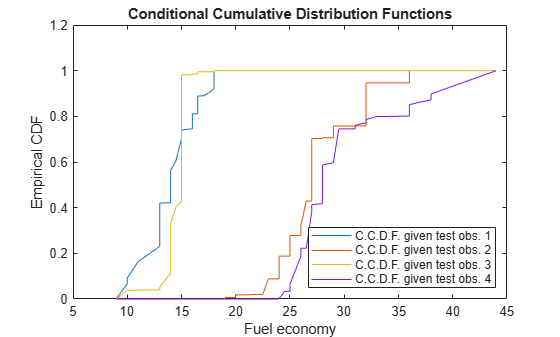# quantilePredict

Class: TreeBagger

Predict response quantile using bag of regression trees

## Syntax

``YFit = quantilePredict(Mdl,X)``
``YFit = quantilePredict(Mdl,X,Name,Value)``
``````[YFit,YW] = quantilePredict(___)``````

## Description

example

``YFit = quantilePredict(Mdl,X)` returns a vector of medians of the predicted responses at `X`, a table or matrix of predictor data, and using the bag of regression trees `Mdl`. `Mdl` must be a `TreeBagger` model object.`

example

``YFit = quantilePredict(Mdl,X,Name,Value)` uses additional options specified by one or more `Name,Value` pair arguments. For example, specify quantile probabilities or which trees to include for quantile estimation.`

example

``````[YFit,YW] = quantilePredict(___)``` also returns a sparse matrix of response weights.```

## Input Arguments

expand all

Bag of regression trees, specified as a `TreeBagger` model object created by `TreeBagger`. The value of `Mdl.Method` must be `regression`.

Predictor data used to estimate quantiles, specified as a numeric matrix or table.

Each row of `X` corresponds to one observation, and each column corresponds to one variable.

• For a numeric matrix:

• The variables making up the columns of `X` must have the same order as the predictor variables that trained `Mdl`.

• If you trained `Mdl` using a table (for example, `Tbl`), then `X` can be a numeric matrix if `Tbl` contains all numeric predictor variables. If `Tbl` contains heterogeneous predictor variables (for example, numeric and categorical data types) and `X` is a numeric matrix, then `quantilePredict` throws an error.

• For a table:

• `quantilePredict` does not support multicolumn variables and cell arrays other than cell arrays of character vectors.

• If you trained `Mdl` using a table (for example, `Tbl`), then all predictor variables in `X` must have the same variable names and data types as those variables that trained `Mdl` (stored in `Mdl.PredictorNames`). However, the column order of `X` does not need to correspond to the column order of `Tbl`. `Tbl` and `X` can contain additional variables (response variables, observation weights, etc.), but `quantilePredict` ignores them.

• If you trained `Mdl` using a numeric matrix, then the predictor names in `Mdl.PredictorNames` and corresponding predictor variable names in `X` must be the same. To specify predictor names during training, see the `PredictorNames` name-value pair argument of `TreeBagger`. All predictor variables in `X` must be numeric vectors. `X` can contain additional variables (response variables, observation weights, etc.), but `quantilePredict` ignores them.

Data Types: `table` | `double` | `single`

### Name-Value Arguments

Specify optional comma-separated pairs of `Name,Value` arguments. `Name` is the argument name and `Value` is the corresponding value. `Name` must appear inside quotes. You can specify several name and value pair arguments in any order as `Name1,Value1,...,NameN,ValueN`.

Quantile probability, specified as the comma-separated pair consisting of `'Quantile'` and a numeric vector containing values in the interval [0,1]. For each observation (row) in `X`, `quantilePredict` returns corresponding quantiles for all probabilities in `Quantile`.

Example: `'Quantile',[0 0.25 0.5 0.75 1]`

Data Types: `single` | `double`

Indices of trees to use in response estimation, specified as the comma-separated pair consisting of `'Trees'` and `'all'` or a numeric vector of positive integers. Indices correspond to the cells of `Mdl.Trees`; each cell therein contains a tree in the ensemble. The maximum value of `Trees` must be less than or equal to the number of trees in the ensemble (`Mdl.NumTrees`).

For `'all'`, `quantilePredict` uses the indices `1:Mdl.NumTrees`.

Example: `'Trees',[1 10 Mdl.NumTrees]`

Data Types: `char` | `string` | `single` | `double`

Weights to attribute to responses from individual trees, specified as the comma-separated pair consisting of `'TreeWeights'` and a numeric vector of `numel(trees)` nonnegative values. `trees` is the value of the `Trees` name-value pair argument.

The default is `ones(size(trees))`.

Data Types: `single` | `double`

Indicators specifying which trees to use to make predictions for each observation, specified as the comma-separated pair consisting of `'UseInstanceForTree'` and an n-by-`Mdl.Trees` logical matrix. n is the number of observations (rows) in `X`. Rows of `UseInstanceForTree` correspond to observations and columns correspond to learners in `Mdl.Trees`. `'all'` indicates to use all trees for all observations when estimating the quantiles.

If `UseInstanceForTree(j,k)` = `true`, then `quantilePredict` uses the tree in `Mdl.Trees(trees(k))` when it predicts the response for the observation `X(j,:)`.

You can estimate the quantile using the response data in `Mdl.Y` directly instead of using the predictions from the random forest by specifying a row composed entirely of `false` values. For example, to estimate the quantile for observation `j` using the response data, and to use the predictions from the random forest for all other observations, specify this matrix:

```UseInstanceForTree = true(size(Mdl.X,2),Mdl.NumTrees); UseInstanceForTree(j,:) = false(1,Mdl.NumTrees); ```

Data Types: `char` | `string` | `logical`

## Output Arguments

expand all

Estimated quantiles, returned as an `n`-by-`numel(tau)` numeric matrix. `n` is the number of observations in `X` (`size(X,1)`) and `tau` is the value of `Quantile`. That is, `YFit(j,k)` is the estimated `100*tau(k)`% percentile of the response distribution given `X(j,:)` and using `Mdl`.

Response weights, returned as an ntrain-by-n sparse matrix. ntrain is the number of responses in the training data (`numel(Mdl.Y)`) and n is the number of observations in `X` (`size(X,1)`).

`quantilePredict` predicts quantiles using linear interpolation of the empirical cumulative distribution function (C.D.F.). For a particular observation, you can use its response weights to estimate quantiles using alternative methods, such as approximating the C.D.F. using kernel smoothing.

Note

`quantilePredict` derives response weights by passing an observation through the trees in the ensemble. If you specify `UseInstanceForTree` and you compose row `j` entirely of `false` values, then `YW(:,j)` = `Mdl.W` instead, that is, the observation weights.

## Examples

expand all

Load the `carsmall` data set. Consider a model that predicts the fuel economy of a car given its engine displacement.

`load carsmall`

Train an ensemble of bagged regression trees using the entire data set. Specify 100 weak learners.

```rng(1); % For reproducibility Mdl = TreeBagger(100,Displacement,MPG,'Method','regression');```

`Mdl` is a `TreeBagger` ensemble.

Perform quantile regression to predict the median MPG for all sorted training observations.

`medianMPG = quantilePredict(Mdl,sort(Displacement));`

`medianMPG` is an `n`-by-1 numeric vector of medians corresponding to the conditional distribution of the response given the sorted observations in `Displacement`. `n` is the number of observations in `Displacement`.

Plot the observations and the estimated medians on the same figure. Compare the median and mean responses.

```meanMPG = predict(Mdl,sort(Displacement)); figure; plot(Displacement,MPG,'k.'); hold on plot(sort(Displacement),medianMPG); plot(sort(Displacement),meanMPG,'r--'); ylabel('Fuel economy'); xlabel('Engine displacement'); legend('Data','Median','Mean'); hold off;```Load the `carsmall` data set. Consider a model that predicts the fuel economy of a car given its engine displacement.

`load carsmall`

Train an ensemble of bagged regression trees using the entire data set. Specify 100 weak learners.

```rng(1); % For reproducibility Mdl = TreeBagger(100,Displacement,MPG,'Method','regression');```

Perform quantile regression to predict the 2.5% and 97.5% percentiles for ten equally-spaced engine displacements between the minimum and maximum in-sample displacement.

```predX = linspace(min(Displacement),max(Displacement),10)'; quantPredInts = quantilePredict(Mdl,predX,'Quantile',[0.025,0.975]);```

`quantPredInts` is a 10-by-2 numeric matrix of prediction intervals corresponding to the observations in `predX`. The first column contains the 2.5% percentiles and the second column contains the 97.5% percentiles.

Plot the observations and the estimated medians on the same figure. Compare the percentile prediction intervals and the 95% prediction intervals assuming the conditional distribution of `MPG` is Gaussian.

```[meanMPG,steMeanMPG] = predict(Mdl,predX); stndPredInts = meanMPG + [-1 1]*norminv(0.975).*steMeanMPG; figure; h1 = plot(Displacement,MPG,'k.'); hold on h2 = plot(predX,quantPredInts,'b'); h3 = plot(predX,stndPredInts,'r--'); ylabel('Fuel economy'); xlabel('Engine displacement'); legend([h1,h2(1),h3(1)],{'Data','95% percentile prediction intervals',... '95% Gaussian prediction intervals'}); hold off;```Load the `carsmall` data set. Consider a model that predicts the fuel economy of a car given its engine displacement.

`load carsmall`

Train an ensemble of bagged regression trees using the entire data set. Specify 100 weak learners.

```rng(1); % For reproducibility Mdl = TreeBagger(100,Displacement,MPG,'Method','regression');```

Estimate the response weights for a random sample of four training observations. Plot the training sample and identify the chosen observations.

```[predX,idx] = datasample(Mdl.X,4); [~,YW] = quantilePredict(Mdl,predX); n = numel(Mdl.Y); figure; plot(Mdl.X,Mdl.Y,'o'); hold on plot(predX,Mdl.Y(idx),'*','MarkerSize',10); text(predX-10,Mdl.Y(idx)+1.5,{'obs. 1' 'obs. 2' 'obs. 3' 'obs. 4'}); legend('Training Data','Chosen Observations'); xlabel('Engine displacement') ylabel('Fuel economy') hold off````YW` is an `n`-by-4 sparse matrix containing the response weights. Columns correspond to test observations and rows correspond to responses in the training sample. Response weights are independent of the specified quantile probability.

Estimate the conditional cumulative distribution function (C.C.D.F.) of the responses by:

1. Sorting the responses is ascending order, and then sorting the response weights using the indices induced by sorting the responses.

2. Computing the cumulative sums over each column of the sorted response weights.

```[sortY,sortIdx] = sort(Mdl.Y); cpdf = full(YW(sortIdx,:)); ccdf = cumsum(cpdf);```

`ccdf(:,j)` is the empirical C.C.D.F. of the response given test observation `j`.

Plot the four empirical C.C.D.F. in the same figure.

```figure; plot(sortY,ccdf); legend('C.C.D.F. given test obs. 1','C.C.D.F. given test obs. 2',... 'C.C.D.F. given test obs. 3','C.C.D.F. given test obs. 4',... 'Location','SouthEast') title('Conditional Cumulative Distribution Functions') xlabel('Fuel economy') ylabel('Empirical CDF')```expand all

## Tips

`quantilePredict` estimates the conditional distribution of the response using the training data every time you call it. To predict many quantiles efficiently, or quantiles for many observations efficiently, you should pass `X` as a matrix or table of observations and specify all quantiles in a vector using the `Quantile` name-value pair argument. That is, avoid calling `quantilePredict` within a loop.

## Algorithms

• `TreeBagger` grows a random forest of regression trees using the training data. Then, to implement quantile random forest, `quantilePredict` predicts quantiles using the empirical conditional distribution of the response given an observation from the predictor variables. To obtain the empirical conditional distribution of the response:

1. `quantilePredict` passes all the training observations in `Mdl.X` through all the trees in the ensemble, and stores the leaf nodes of which the training observations are members.

2. `quantilePredict` similarly passes each observation in `X` through all the trees in the ensemble.

3. For each observation in `X`, `quantilePredict`:

1. Estimates the conditional distribution of the response by computing response weights for each tree.

2. For observation k in `X`, aggregates the conditional distributions for the entire ensemble:

`$\stackrel{^}{F}\left(y|X={x}_{k}\right)=\sum _{j=1}^{n}\sum _{t=1}^{T}\frac{1}{T}{w}_{tj}\left({x}_{k}\right)I\left\{{Y}_{j}\le y\right\}.$`

n is the number of training observations (`size(Y,1)`) and T is the number of trees in the ensemble (`Mdl.NumTrees`).

4. For observation k in `X`, the τ quantile or, equivalently, the 100τ% percentile, is ${Q}_{\tau }\left({x}_{k}\right)=\mathrm{inf}\left\{y:\stackrel{^}{F}\left(y|X={x}_{k}\right)\ge \tau \right\}.$

• This process describes how `quantilePredict` uses all specified weights.

1. For all training observations j = 1,...,n and all chosen trees t = 1,...,T,

`quantilePredict` attributes the product vtj = btjwj,obs to training observation j (stored in `Mdl.X(j,:)` and `Mdl.Y(j)`). btj is the number of times observation j is in the bootstrap sample for tree t. wj,obs is the observation weight in `Mdl.W(j)`.

2. For each chosen tree, `quantilePredict` identifies the leaves in which each training observation falls. Let St(xj) be the set of all observations contained in the leaf of tree t of which observation j is a member.

3. For each chosen tree, `quantilePredict` normalizes all weights within a particular leaf to sum to 1, that is,

`${v}_{tj}^{\ast }=\frac{{v}_{tj}}{\sum _{i\in {S}_{t}\left({x}_{j}\right)}{v}_{ti}}.$`

4. For each training observation and tree, `quantilePredict` incorporates tree weights (wt,tree) specified by `TreeWeights`, that is, w*tj,tree = wt,treevtj*Trees not chosen for prediction have 0 weight.

5. For all test observations k = 1,...,K in `X` and all chosen trees t = 1,...,T`quantilePredict` predicts the unique leaves in which the observations fall, and then identifies all training observations within the predicted leaves. `quantilePredict` attributes the weight utj such that

6. `quantilePredict` sums the weights over all chosen trees, that is,

`${u}_{j}=\sum _{t=1}^{T}{u}_{tj}.$`

7. `quantilePredict` creates response weights by normalizing the weights so that they sum to 1, that is,

`${w}_{j}^{\ast }=\frac{{u}_{j}}{{\sum }_{j=1}^{n}{u}_{j}}.$`

## References

 Breiman, L. "Random Forests." Machine Learning 45, pp. 5–32, 2001.

 Meinshausen, N. “Quantile Regression Forests.” Journal of Machine Learning Research, Vol. 7, 2006, pp. 983–999.